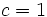# Derived length is logarithmically bounded by nilpotency class

## Statement

Let$G$ be a nilpotent group and let$c$ be the nilpotency class of$G$.Then,$G$ is a solvable group, and if$\ell$ denotes the derived length of$G$, we have:$\ell \le \log_2 c + 1$.

## Facts used

1. Second half of lower central series of nilpotent group comprises abelian groups: If$G$ is nilpotent of class$c$, and$\gamma_k(G)$ denotes the$k^{th}$ term of the lower central series of$G$, then$\gamma_k(G)$ is abelian for$k \ge (c + 1)/2$.

## Proof

We prove this by induction on the nilpotence class. Note that the statement is true when$c = 1$ or$c = 2$.

Given: A finite nilpotent group$G$ of class$c$.

To prove: The derived length of$G$ is at most$\log_2 c + 1$.

Proof: Let$k$ be the smallest positive integer greater than or equal to$(c + 1)/2$. In other words, either$k = (c+1)/2$ or$k = c/2 + 1$, depending on the parity of$c$. Then,$\gamma_k(G)$ is an abelian group, and$G/\gamma_k(G)$ is a group of class$k - 1$, which is at most$c/2$.

By the induction assumption, we have:$\ell(G/\gamma_k(G)) \le \log_2(k) + 1 \le \log_2 (c/2) + 1 = \log_2 c$.

Thus,$G$ has an abelian normal subgroup such that the derived length of the quotient is at most$\log_2 c$. This yields that the derived length of$G$ is at most$\log_2 c + 1$.